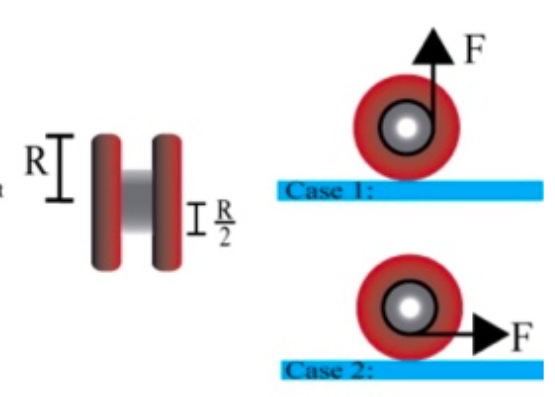# Problem: A yoyo is constructed by attaching three uniform, solid disks along their central axes as shown. The two outer disks are identical, each with mass M = 56 g, radius R = 3.3 cm, and moment of inertia 1/2MR2. The central, smaller disk has mass M/2 and radius R/2. A light, flexible string of negligible mass is wrapped counterclockwise around the central disk of the yoyo. The yoyo is then placed on a horizontal tabletop and the string is gently pulled with a constant force F = 0.375 N. The tension in the string is not sufficient to cause the yoyo to leave the tabletop. In this problem consider the two cases show. In Case 1 the string is pulled straight up, perpendicular to the tabletop. In Case 2 the string is pulled horizontally, parallel to the tabletop. In both cases the yoyo rolls without slipping. Part (a) What is the moment of inertia Icm about the central axis (i.e. the axis perpendicular to the circular face) of the yoyo, in kq-m2? Part (b) In both cases shown, what is the magnitude of the torque t exerted by the string about the contact point of the yo-yo with the table, in N.m? Part (c) What is the moment of inertia of the yo-yo about the contact point with the table, in kg-m? (d) What is the magnitude of the linear acceleration of the center of mass of the yoyo the moment the string becomes taut, in m/s2

###### FREE Expert Solution

Torque:

$\overline{){\mathbf{\tau }}{\mathbf{=}}{\mathbf{r}}{\mathbf{×}}{\mathbf{F}}}$

96% (423 ratings)###### Problem Details

A yoyo is constructed by attaching three uniform, solid disks along their central axes as shown. The two outer disks are identical, each with mass M = 56 g, radius R = 3.3 cm, and moment of inertia 1/2MR2. The central, smaller disk has mass M/2 and radius R/2. A light, flexible string of negligible mass is wrapped counterclockwise around the central disk of the yoyo. The yoyo is then placed on a horizontal tabletop and the string is gently pulled with a constant force F = 0.375 N. The tension in the string is not sufficient to cause the yoyo to leave the tabletop. In this problem consider the two cases show. In Case 1 the string is pulled straight up, perpendicular to the tabletop. In Case 2 the string is pulled horizontally, parallel to the tabletop. In both cases the yoyo rolls without slipping.Part (a) What is the moment of inertia Icm about the central axis (i.e. the axis perpendicular to the circular face) of the yoyo, in kq-m2

Part (b) In both cases shown, what is the magnitude of the torque t exerted by the string about the contact point of the yo-yo with the table, in N.m? Part (c) What is the moment of inertia of the yo-yo about the contact point with the table, in kg-m? (d) What is the magnitude of the linear acceleration of the center of mass of the yoyo the moment the string becomes taut, in m/s2